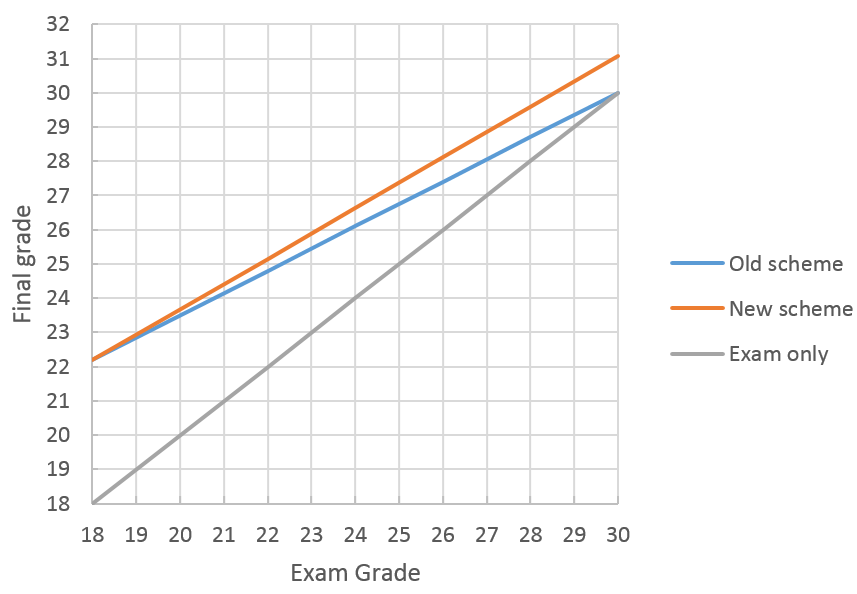by Simon Razniewski -
Number of replies: 0

Dear students,

Based on your feedback about the calculation of the final mark from assignments and exam the formula has been modified. Given the following abbreviations,

AW = weight of assignments that are at least as good as the exam grade, maximum is 0.35
AG = average grade of the assignments that are at least as good as the exam grade